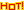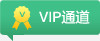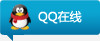• 南加州
• 北加州
• 纽约州
• 麻省
• 内华达
• 华盛顿州
• 华盛顿特区
• 马里兰
• 弗吉尼亚
• 新泽西州
• 北卡罗来纳州
• 佛罗里达州
• 佐治亚州
• 德克萨斯州
• 特拉华州
• 亚利桑那州
• 伊利诺伊州
• 印第安纳州
• 夏威夷州
• 威斯康星州
• 内布拉斯加州
• 密苏里州
• 罗德岛
• 佛蒙特州
• 俄勒冈州
• 宾夕法尼亚州
• 田纳西州
• 新墨西哥州
• 南卡罗来纳州
• 科罗拉多
• 密歇根州
• 新罕布什尔州
• 明尼苏达州
• 俄亥俄州
• 爱达荷州
• 康涅狄格州
• 路易斯安娜州
• 爱荷华
• 犹他州
• 蒙大拿州
• 俄克拉荷马州
• 阿拉巴马州
• 堪萨斯州
• 阿肯色州
• 北达科他州
•亚凯迪亚 Arcadia天普市 Temple City
:q尔湾 Irvine
:q
:q
:q
:q
:q
:q
:q
•帕罗奥图 Palo Alto圣荷西 San Jose
:q
:q
:q
:q
:q
:q
:q
:q
:q
:q
:q
:q
:q
:q
:q
:q
:q
:q
• :q
:q
:q
:q曼哈顿 Manhattan纽约市 New York
:q
:q
:q
•波士顿 Boston
:q昆西 Quincy
:q
:q
:q
:q
:q
:q
• :q
:q
• :q
:q
:q
:q
:q
• :q
• :q
:q
:q
:q
• :q
:q
:q
:q
:q
:q
:q
:q
:q
• :q
:q
:q
:q
:q
:q
:q
:q
:q
:q
:q
:q
• :q
:q
:q
:q
:q
:q
:q
:q
:q
• :q
:q
:q
:q
:q
:q
:q
:q
:q
:q
:q
:q
:q
:q
:q
:q
:q
:q
:q
:q
:q
:q
:q
• :q
:q
:q
:q
:q
:q
:q
:q
:q
•达拉斯 Dallas
:q休斯顿大都会市区 Houston
:q
:q
:q
:q
:q
• :q
• :q
:q
:q
:q
• :q
:q
:q
:q
:q
:q
• :q
:q
:q
• :q
• :q
:q
:q
:q
:q
:q
:q
:q
• :q
• :q
:q
• :q
:q
:q
:q
:q
• :q
:q
• :q
:q
:q
:q
:q
:q
• :q
:q
:q
:q
:q
:q
:q
:q
:q
:q
:q
:q
• :q
:q
:q
:q
• :q
• :q
:q
:q
:q
:q
:q
:q
:q
• :q
:q
:q
:q
:q
:q
:q
• :q
:q
:q
:q
• :q
:q
• :q
:q
:q
:q
• :q
:q
:q
:q
:q
:q
:q
• :q
• :q
:q
:q
:q
:q
• :q
:q
• :q
• :q
:q
:q
• :q
:q
:q
:q
:q
:q
• :q
:q
• :q
:q
:q
:q
• :q
:q曼哈顿 Manhattan
:q
:q
:q
:q
:q
• :q
:q
:q
• :q
:qcontact@meifang8.com

4001-678-009

+1 858-429-5888